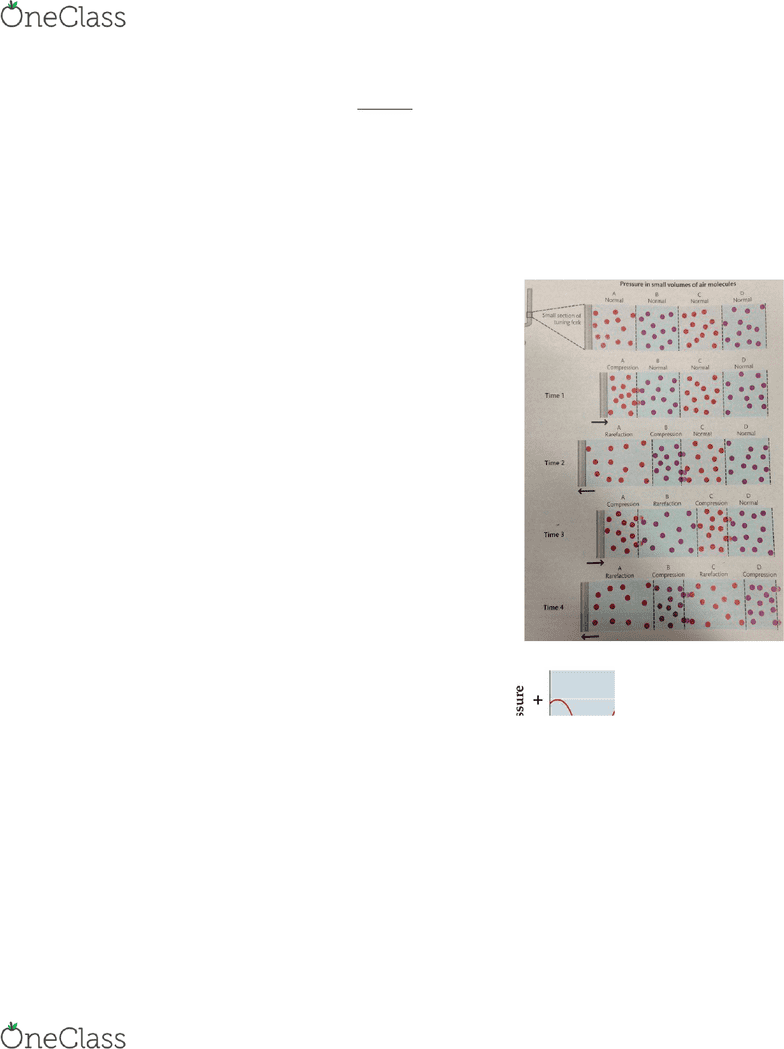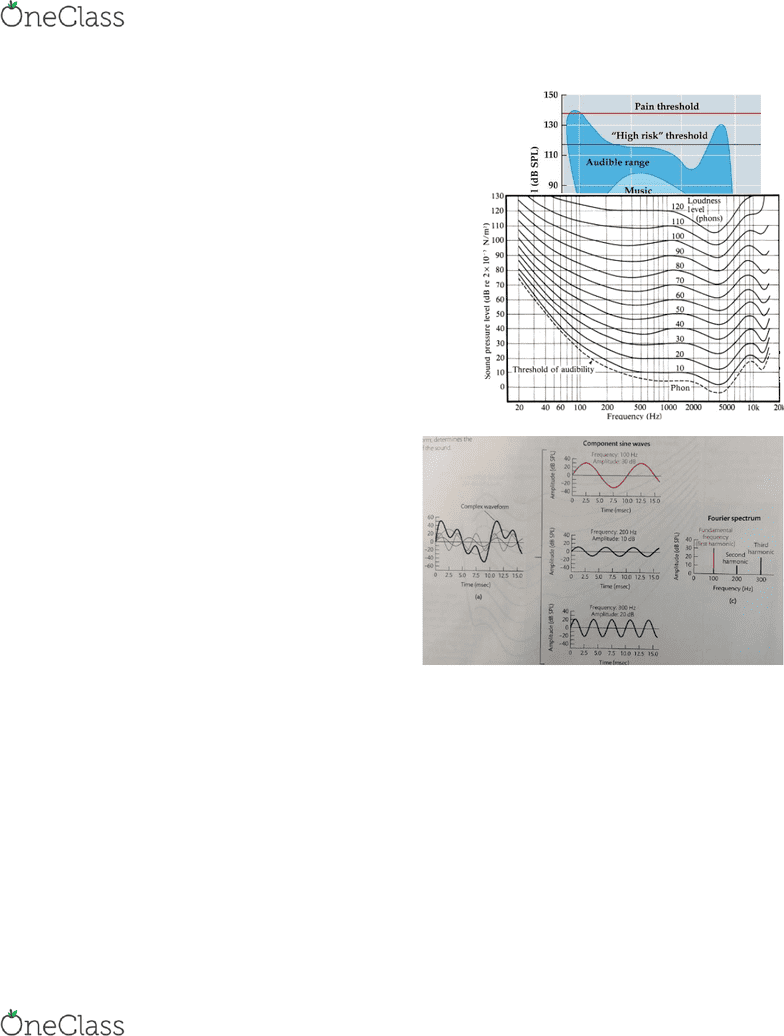Textbook Notes (270,000)
CA (160,000)
Concordia (1,000)
PSYC (200)
Chapter 9

# PSYC 363 Chapter Notes - Chapter 9: Tuning Fork, Inverse-Square Law, Sound

Department
Psychology
Course Code
PSYC 363
Professor
Michael Lantz
Chapter
9

This preview shows pages 1-3. to view the full 13 pages of the document.Chapter 9: Sound and the Ears
SOUND
Sound
1. Changes in pressure over time.
Sources of Sound
1. Sound is initiated by:
a) Movement that disturbs air molecules:
i. Results in changes in air pressure that propagate outward from the source.
2. Tuning fork example:
a) Procedure:
i. Tuning fork is struck.
ii. Tuning fork is moved to the right:
- Air molecules get pushed to the right, which push on the
molecules to their right, and so on:
oResults in an increase in air pressure (i.e. air
molecules are compressed) in the space to the right of the
source.
iii. Tuning fork moves back to the left:
- Makes more room for the air molecules rebounding
from the collision:
oResults in a decrease in air pressure in that space.
- The effects of the initial collision continue to
propagate to the right.
iv. Sound waves are detected at great distances from
their source.
3. Sound waves:
a) Waves of pressure changes in air cause by the vibrations
of a source.
4. Graph of variations in air pressure:
a) Illustrates how air pressure varies at a fixed location next the
tuning fork (source).
b) Horizontal line depicts:
i. Normal atmospheric pressure:
- Air pressure in the absence of sound.
c) One cycle in a sound wave:
i. A repeating segment of air pressure changes.
d) Inverse square law:
i. The falloff in sound energy with distance:
- Energy of the sound decreases in proportion to the square of the distance from the
source (i.e. distant sound is quieter).
Physical and Perceptual Dimensions of Sound
1. Three physical dimensions of sound:
a) Frequency:
find more resources at oneclass.com
find more resources at oneclass.com

Only pages 1-3 are available for preview. Some parts have been intentionally blurred.Chapter 9: Sound and the Ears
i. Related to pitch.
b) Amplitude:
i. Related to loudness.
c) Waveform:
i. Related to timber (quality of sound).
2. Periotic sound waves:
a) Waves in which the cycles of compression and rarefaction repeat in regular, or
periodic, fashion.
b) Simplest periotic sound waves:
i. Pure tone:
- A sound wave in which air pressure changes over time according to a
mathematical formula called a sine wave (or sinusoid).
3. Frequency and Pitch:
a) Frequency of a pure tone:
i. The physical dimension of sound that is related to the perceptual dimension of
pitch.
ii. Expressed in hertz:
- The number of cycles per second of a periodic sound wave.
- The physical unit used to measure frequency.
b) Pitch:
i. The perceptual dimension of sound that corresponds to the physical dimension of
frequency.
ii. The perceived highness of lowness of sound.
4. Amplitude and Loudness:
a) Amplitude of a pure tone:
i. The difference between the maximum and minimum sound pressure in a sound
wave.
ii. The physical dimension of sound that is related to the perceptual dimension of
loudness.
iii. Expressed in decibels (dB):
- A physical unit used to measure sound amplitude.
- Logarithmically related to sound pressure (measured in micropascales):
oThe decibel scale is not linear:
A 6 dB increase requires double the amount of energy.
Example:
1) 20 dB = 200 micropascales pressure.
2) 40 dB = 2000 micropascales pressure.
b) Loudness:
i. The perceptual dimension of sound that is related to the physical dimension of
amplitude (i.e. the inverse of amplitude).
ii. How intense or quiet a sound seems.
5. Audible curve (the absolute threshold for hearing):
a) Threshold:
i. Recall Absolute Threshold:
find more resources at oneclass.com
find more resources at oneclass.com

Only pages 1-3 are available for preview. Some parts have been intentionally blurred.Chapter 9: Sound and the Ears
- The intensity of the least intense stimulus that can be
detected by the modality.
ii. The threshold of hearing:
- Depends on the sound’s frequency (i.e. wavelength of
the sound).
b) The audible curve:
i. A curve showing the minimum amplitude at
which sound can be detected at each frequency:
- Auditory sensitivity is maximal in the middle
range (i.e. frequencies of human speech).
6. Equal Loudness Contours
a) Equal Loudness contours:
i. A curve showing the amplitude of tones at
different frequencies that sound about equally
loud.
ii. Shows us how loudness varies with frequency
iii. The hearing of humans is best when frequencies
are between 500 and 5000 Hz.
7. Waveform and Timber:
a) Most periotic sounds (e.g., vocal cords,
clarinets, etc.) consist of multiple pure
i. The waveforms of such sounds have a
more complex shape than a sine
wave:
- These waveforms are broken down
into sine waves with the Fourier
Analysis.
b) The Fourier Analysis:
i. A mathematical procedure for
decomposing a complex waveform
into a collection of sine waves with various frequencies and amplitudes.
c) The Fourier Spectrum:
i. A depiction of the amplitudes at all frequencies that make up a complex
waveform.
d) The Fundamental Frequency:
i. The frequency of the lowest-frequency component of a complex waveform:
- Determines the perceived pitch of the sound
e) Harmonic:
i. A component frequency of a complex waveform that is an integer multiple of the
fundamental frequency:
- The first harmonic is the fundamental frequency.
- The second harmonic is twice the fundamental frequency (i.e. the second-lowest
frequency component).
- Etc.
find more resources at oneclass.com
find more resources at oneclass.com